# explore

#### 2022-01-29

The explore package simplifies Exploratory Data Analysis (EDA). Get faster insights with less code!

There are three ways to use the package:

• Interactive data exploration (univariat, bivariat, multivariat)

• Generate an Automated Report with one line of code. The target can be binary, categorical or numeric.

• Manual exploration using a easy to remember set of tidy functions. Introduces four main verbs. explore() to grafically explore a variable or table, describe() to describe a variable or table, explain_tree() to create a simple decision tree that explains a target. report() to generate an automated report of all variables.

explore package on Github: https://github.com/rolkra/explore

As the explore-functions fits well into the tidyverse, we load the dplyr-package as well.

library(dplyr)
library(explore)

### Interactive data exploration

Explore your dataset (in this case the iris dataset) in one line of code:

explore(iris)

A shiny app is launched, you can inspect individual variable, explore their relation to a target (binary / categorical / numerical), grow a decision tree or create a fully automated report of all variables with a few “mouseclicks”.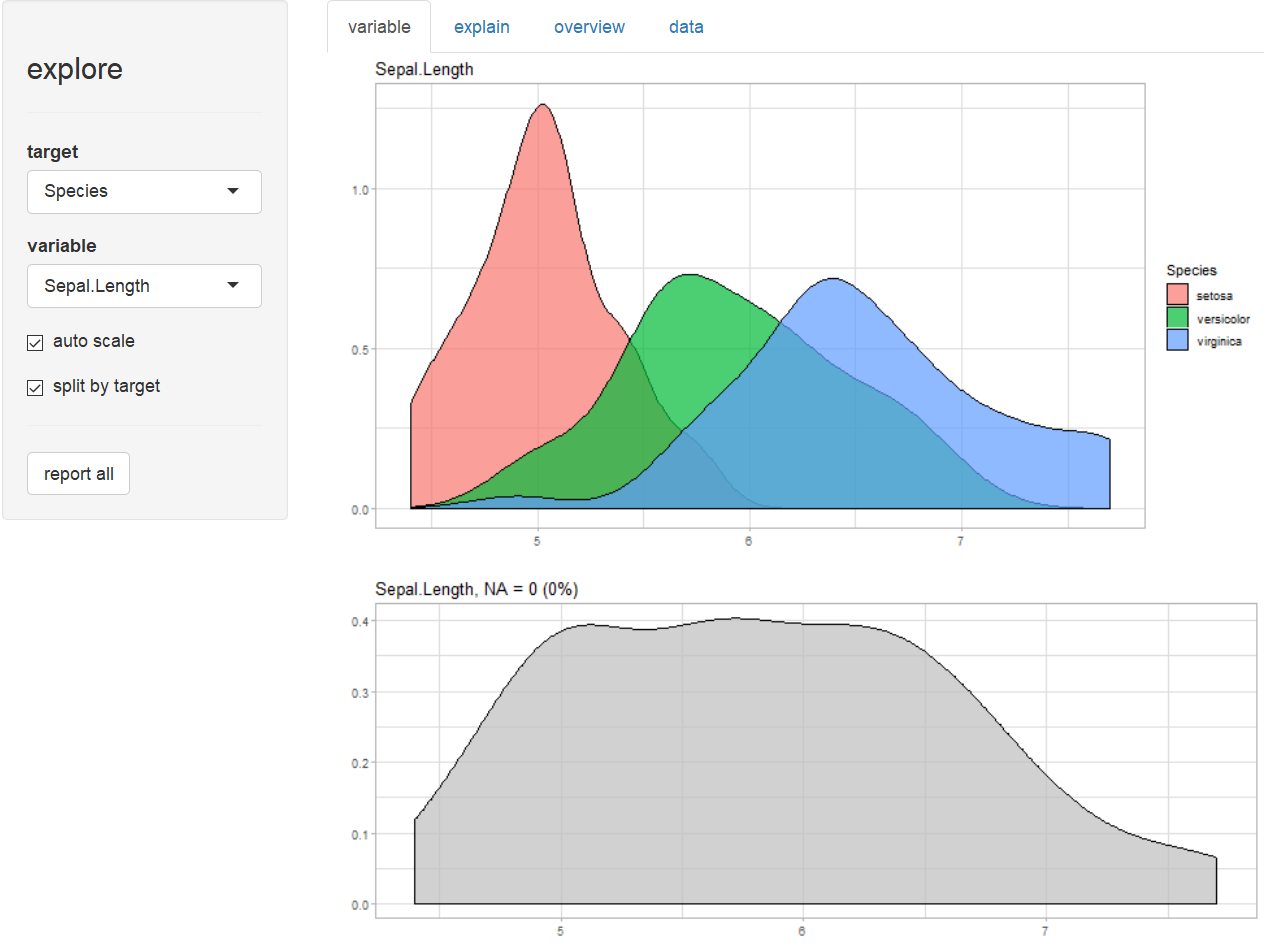You can choose each variable containng as a target, that is binary (0/1, FALSE/TRUE or “no”/“yes”), categorical or numeric.

### Report variables

Create a rich HTML report of all variables with one line of code:

# report of all variables
iris %>% report(output_file = "report.html", output_dir = tempdir())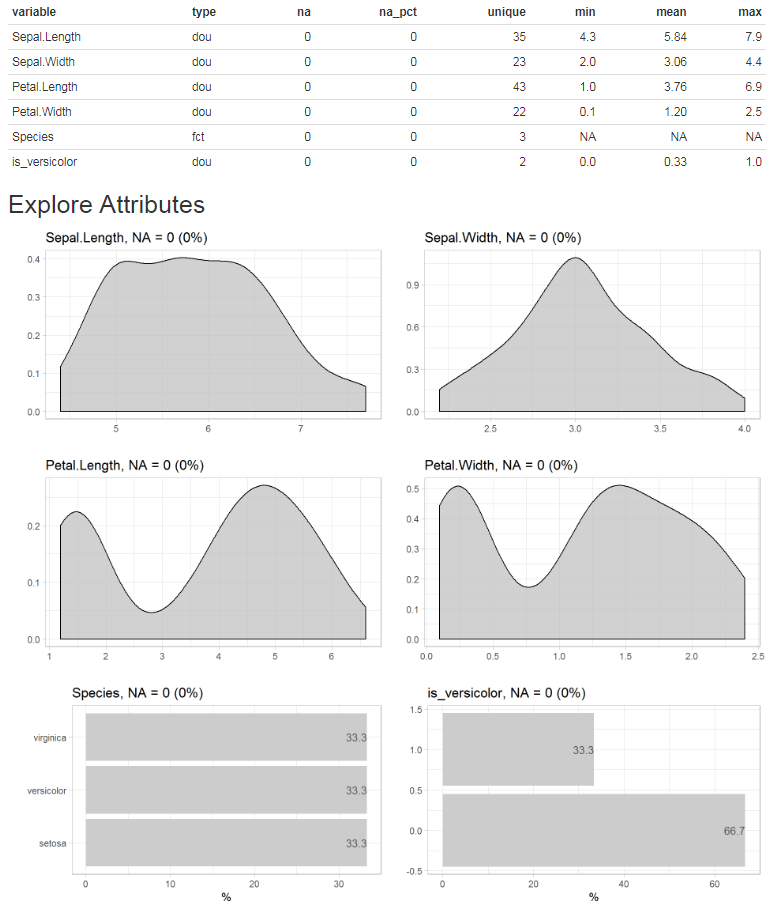Or you can simply add a target and create the report. In this case we use a binary tharget, but a categorical or numerical target would work as well.

# report of all variables and their relationship with a binary target
iris$is_versicolor <- ifelse(iris$Species == "versicolor", 1, 0)
iris %>%
report(output_file = "report.html",
output_dir = tempdir(),
target = is_versicolor)

If you use a binary tharget, the parameter split = FALSE will give you a different view on the data.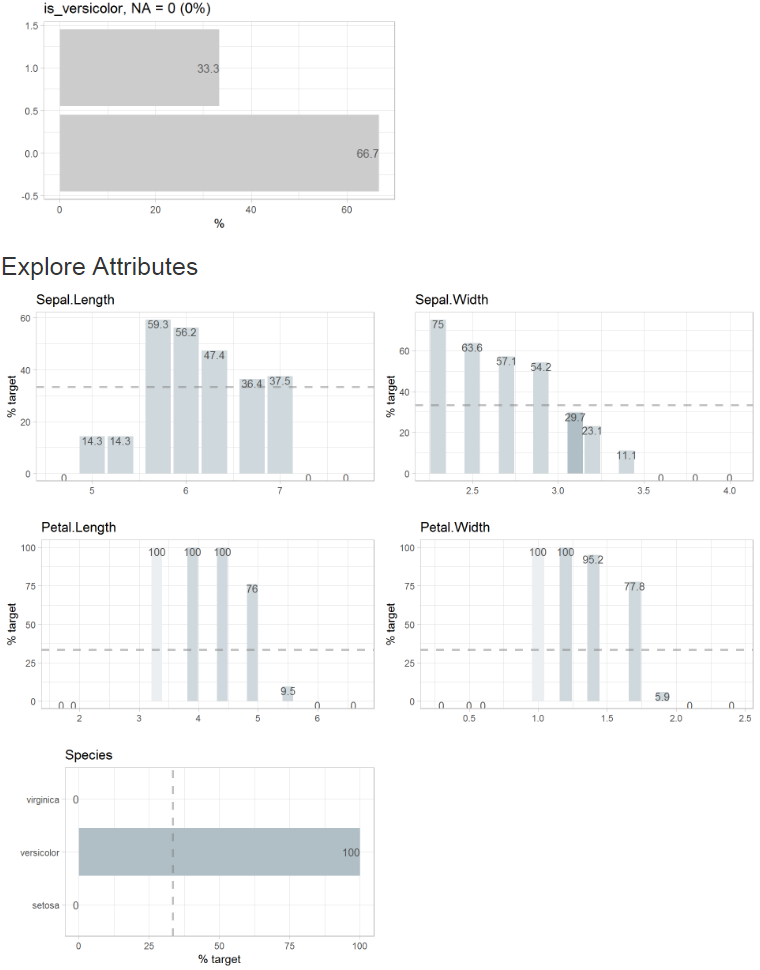### Grow a decision tree

Grow a decision tree with one line of code:

iris %>% explain_tree(target = Species)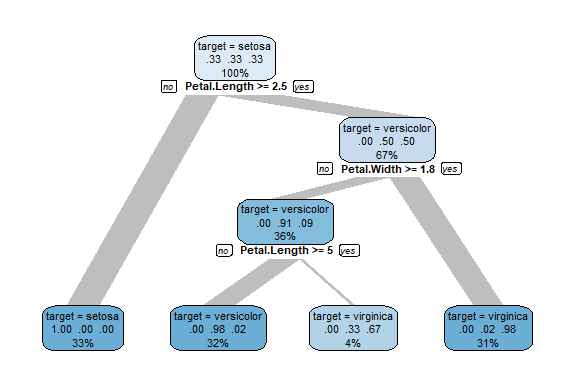You can grow a decision tree with a binary target too.

iris$is_versicolor <- ifelse(iris$Species == "versicolor", 1, 0)
iris %>% select(-Species) %>% explain_tree(target = is_versicolor)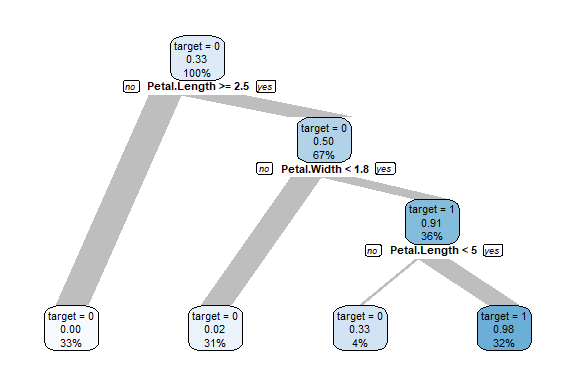Or using a numerical target. The syntax stays the same.

iris %>% explain_tree(target = Sepal.Length)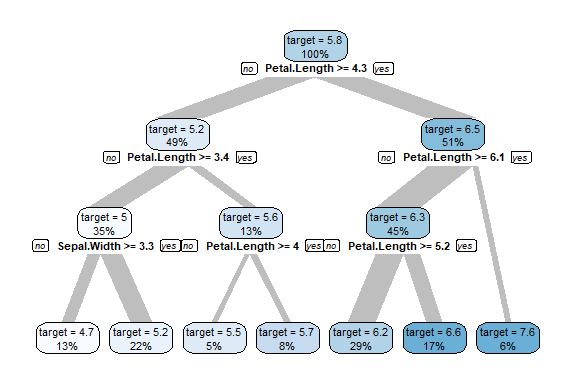You can control the growth of the tree using the parameters maxdepth, minsplit and cp.

### Explore dataset

Explore your table with one line of code to see which type of variables it contains.

iris %>% explore_tbl()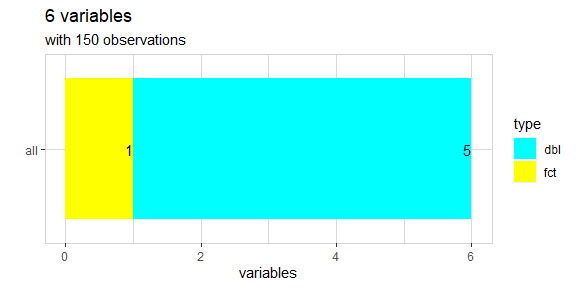You can also use describe_tbl() if you just need the main facts without visualisation.

iris %>% describe_tbl()
#> 150 observations with 6 variables
#> 0 observations containing missings (NA)
#> 0 variables containing missings (NA)
#> 0 variables with no variance

### Explore variables

Explore a variable with one line of code. You don’t have to care if a variable is numerical or categorical.

iris %>% explore(Species)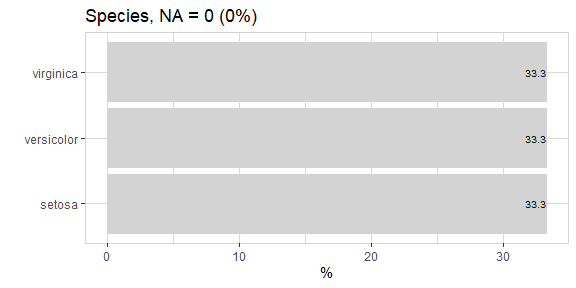iris %>% explore(Sepal.Length)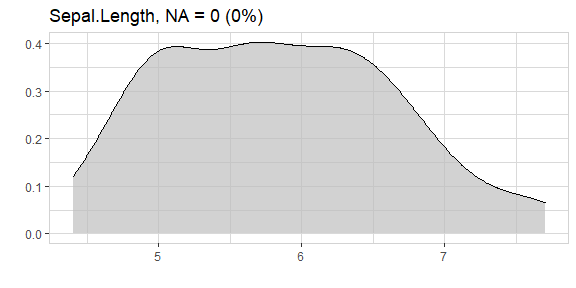### Explore variables with a target

Explore a variable and its relationship with a binary target with one line of code. You don’t have to care if a variable is numerical or categorical.

iris %>% explore(Sepal.Length, target = is_versicolor)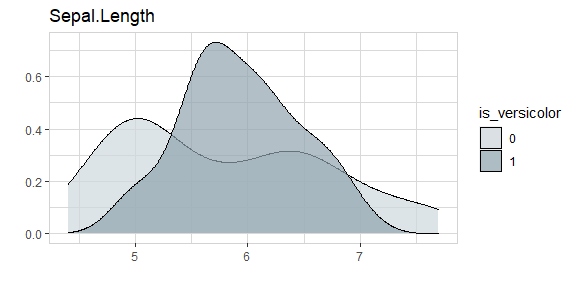Using split = FALSE will change the plot to %target:

iris %>% explore(Sepal.Length, target = is_versicolor, split = FALSE)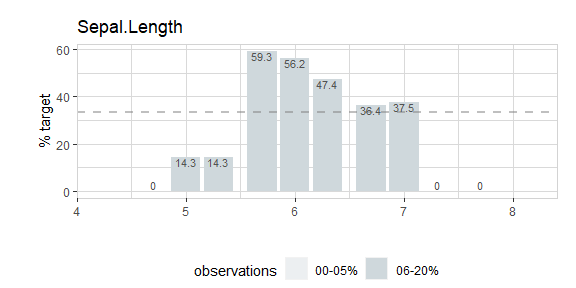The target can have more than two levels:

iris %>% explore(Sepal.Length, target = Species)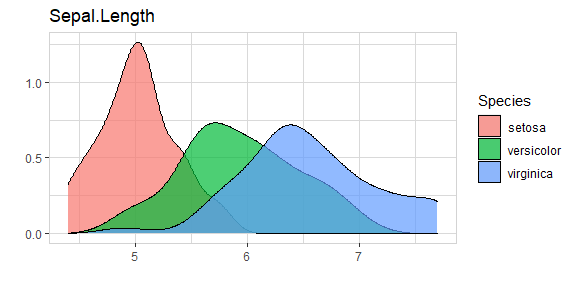Or the target can even be numeric:

iris %>% explore(Sepal.Length, target = Petal.Length)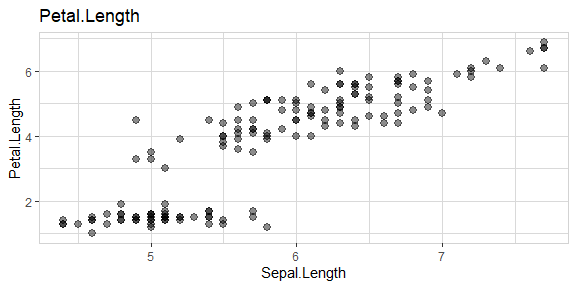### Explore multiple variables

iris %>%
select(Sepal.Length, Sepal.Width) %>%
explore_all()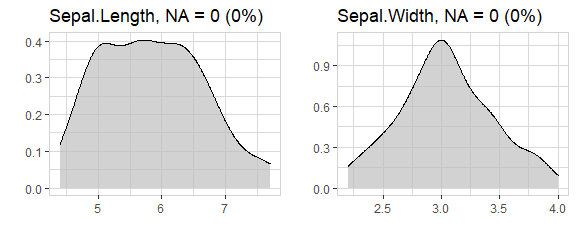iris %>%
select(Sepal.Length, Sepal.Width, is_versicolor) %>%
explore_all(target = is_versicolor)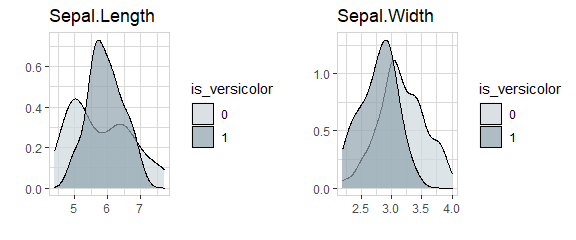iris %>%
select(Sepal.Length, Sepal.Width, is_versicolor) %>%
explore_all(target = is_versicolor, split = FALSE)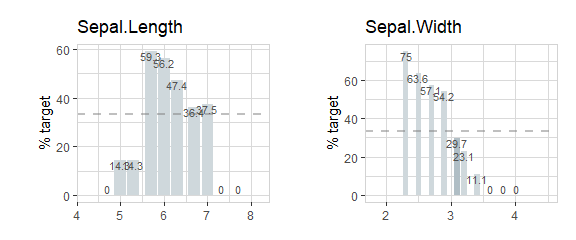iris %>%
select(Sepal.Length, Sepal.Width, Species) %>%
explore_all(target = Species)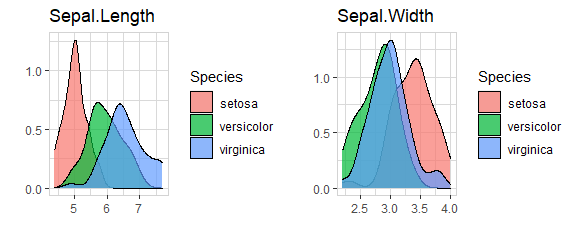iris %>%
select(Sepal.Length, Sepal.Width, Petal.Length) %>%
explore_all(target = Petal.Length)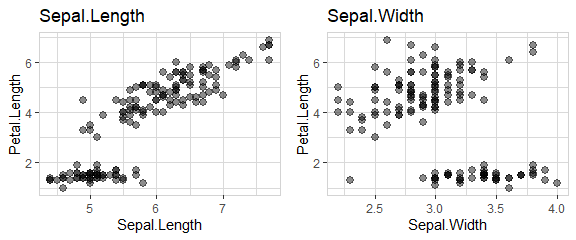data(iris)

To use a high number of variables with explore_all() in a RMarkdown-File, it is necessary to set a meaningful fig.width and fig.height in the junk. The function total_fig_height() helps to automatically set fig.height: fig.height=total_fig_height(iris)

iris %>%
explore_all()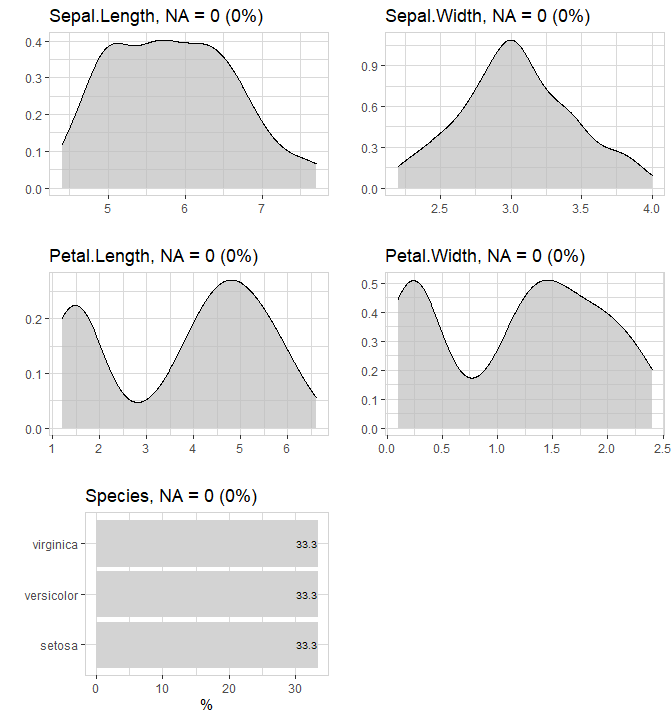If you use a target: fig.height=total_fig_height(iris, var_name_target = "Species")

iris %>% explore_all(target = Species)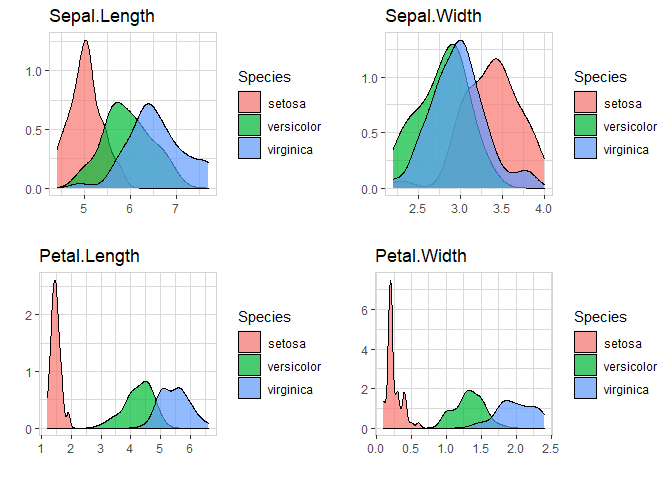You can control total_fig_height() by parameters ncols (number of columns of the plots) and size (height of 1 plot)

### Explore correlation between two variables

Explore correlation between two variables with one line of code:

iris %>% explore(Sepal.Length, Petal.Length)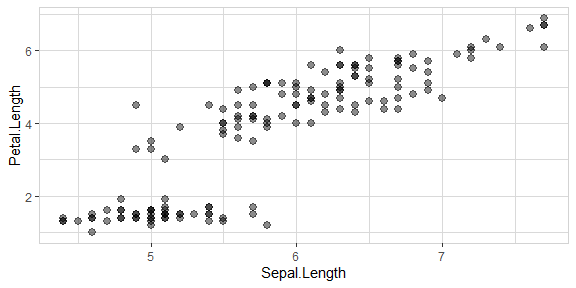You can add a target too:

iris %>% explore(Sepal.Length, Petal.Length, target = Species)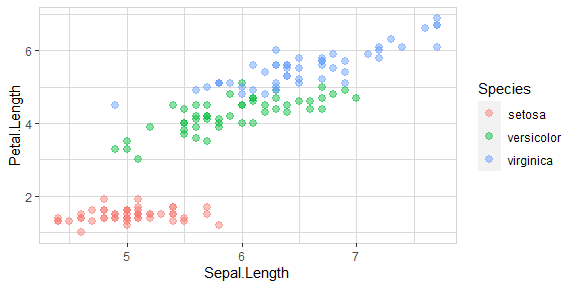### Other options

If you use explore to explore a variable and want to set lower and upper limits for values, you can use the min_val and max_val parameters. All values below min_val will be set to min_val. All values above max_val will be set to max_val.

iris %>% explore(Sepal.Length, min_val = 4.5, max_val = 7)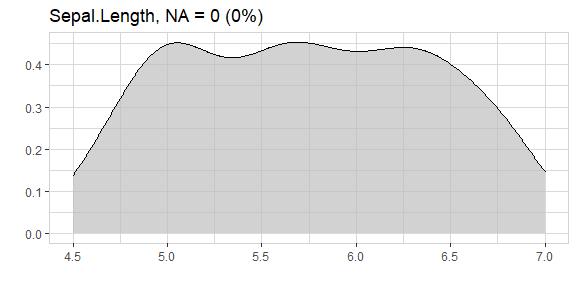explore uses auto-scale by default. To deactivate it use the parameter auto_scale = FALSE

iris %>% explore(Sepal.Length, auto_scale = FALSE)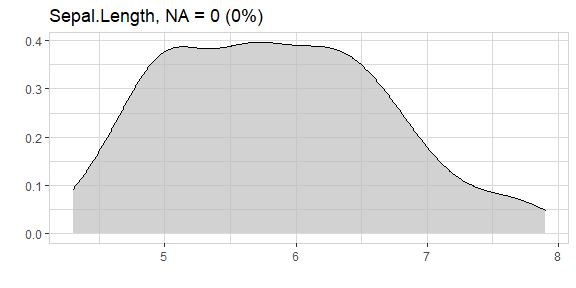### Describing data

Describe your data in one line of code:

iris %>% describe()
#> # A tibble: 5 x 8
#>   variable     type     na na_pct unique   min  mean   max
#>   <chr>        <chr> <int>  <dbl>  <int> <dbl> <dbl> <dbl>
#> 1 Sepal.Length dbl       0      0     35   4.3  5.84   7.9
#> 2 Sepal.Width  dbl       0      0     23   2    3.06   4.4
#> 3 Petal.Length dbl       0      0     43   1    3.76   6.9
#> 4 Petal.Width  dbl       0      0     22   0.1  1.2    2.5
#> 5 Species      fct       0      0      3  NA   NA     NA

The result is a data-frame, where each row is a variable of your data. You can use filter from dplyr for quick checks:

# show all variables that contain less than 5 unique values
iris %>% describe() %>% filter(unique < 5)
#> # A tibble: 1 x 8
#>   variable type     na na_pct unique   min  mean   max
#>   <chr>    <chr> <int>  <dbl>  <int> <dbl> <dbl> <dbl>
#> 1 Species  fct       0      0      3    NA    NA    NA
# show all variables contain NA values
iris %>% describe() %>% filter(na > 0)
#> # A tibble: 0 x 8
#> # ... with 8 variables: variable <chr>, type <chr>, na <int>, na_pct <dbl>,
#> #   unique <int>, min <dbl>, mean <dbl>, max <dbl>

You can use describe for describing variables too. You don’t need to care if a variale is numerical or categorical. The output is a text.

# describe a numerical variable
iris %>% describe(Species)
#> variable = Species
#> type     = factor
#> na       = 0 of 150 (0%)
#> unique   = 3
#>  setosa     = 50 (33.3%)
#>  versicolor = 50 (33.3%)
#>  virginica  = 50 (33.3%)
# describe a categorical variable
iris %>% describe(Sepal.Length)
#> variable = Sepal.Length
#> type     = double
#> na       = 0 of 150 (0%)
#> unique   = 35
#> min|max  = 4.3 | 7.9
#> q05|q95  = 4.6 | 7.255
#> q25|q75  = 5.1 | 6.4
#> median   = 5.8
#> mean     = 5.843333

### Data Dictionary

Create a Data Dictionary of a dataset (Markdown File data_dict.md)

iris %>% data_dict_md(output_dir = tempdir())

Add title, detailed descriptions and change default filename

description <- data.frame(
variable = c("Species"),
description = c("Species of Iris flower"))
data_dict_md(iris,
title = "iris flower data set",
description =  description,
output_file = "data_dict_iris.md",
output_dir = tempdir())

### Basic data cleaning

To clean a variable you can use clean_var. With one line of code you can rename a variable, replace NA-values and set a minimum and maximum for the value.

iris %>%
clean_var(Sepal.Length,
min_val = 4.5,
max_val = 7.0,
na = 5.8,
name = "sepal_length") %>%
describe()
#> # A tibble: 5 x 8
#>   variable     type     na na_pct unique   min  mean   max
#>   <chr>        <chr> <int>  <dbl>  <int> <dbl> <dbl> <dbl>
#> 1 sepal_length dbl       0      0     26   4.5  5.81   7
#> 2 Sepal.Width  dbl       0      0     23   2    3.06   4.4
#> 3 Petal.Length dbl       0      0     43   1    3.76   6.9
#> 4 Petal.Width  dbl       0      0     22   0.1  1.2    2.5
#> 5 Species      fct       0      0      3  NA   NA     NA